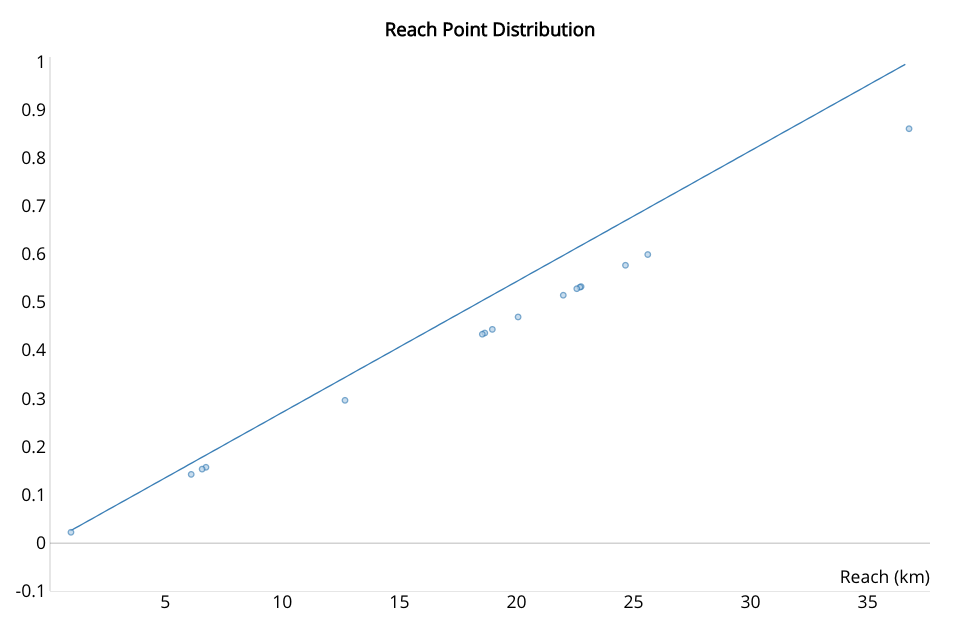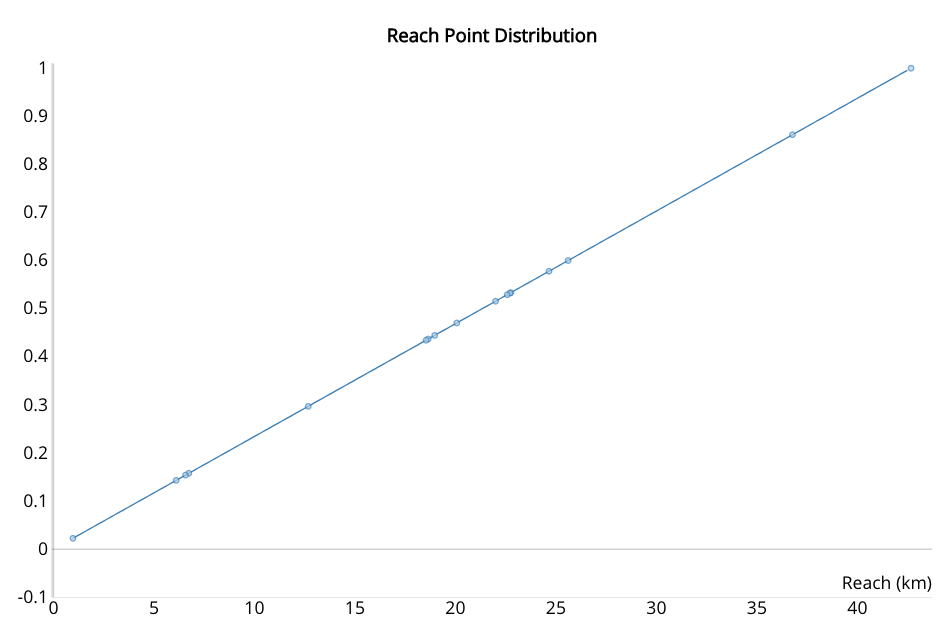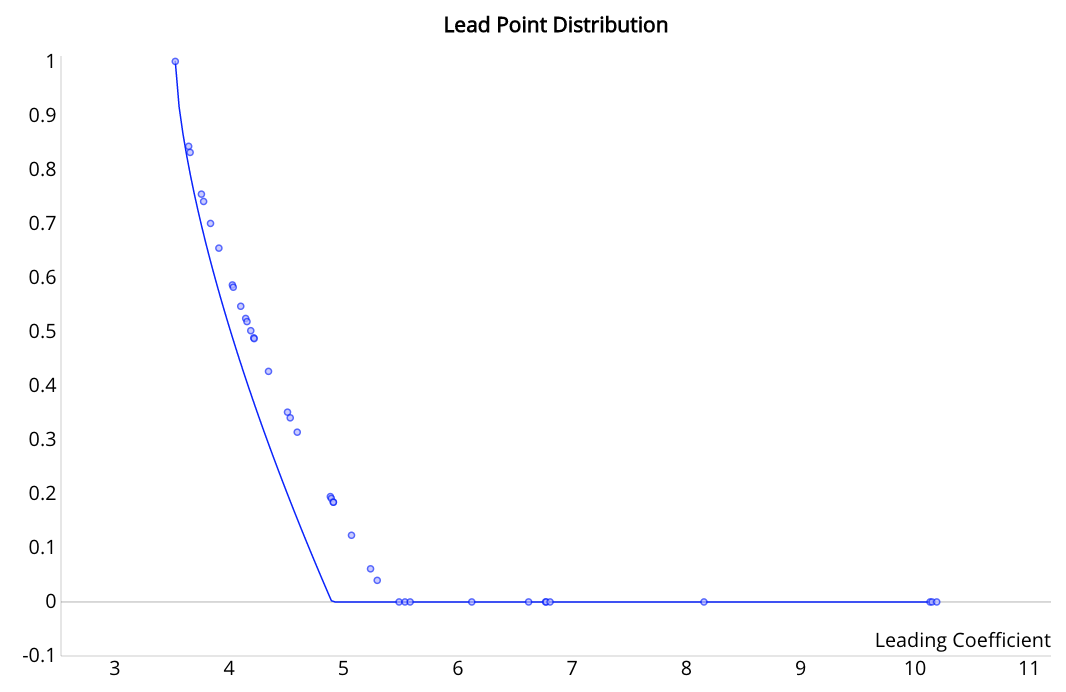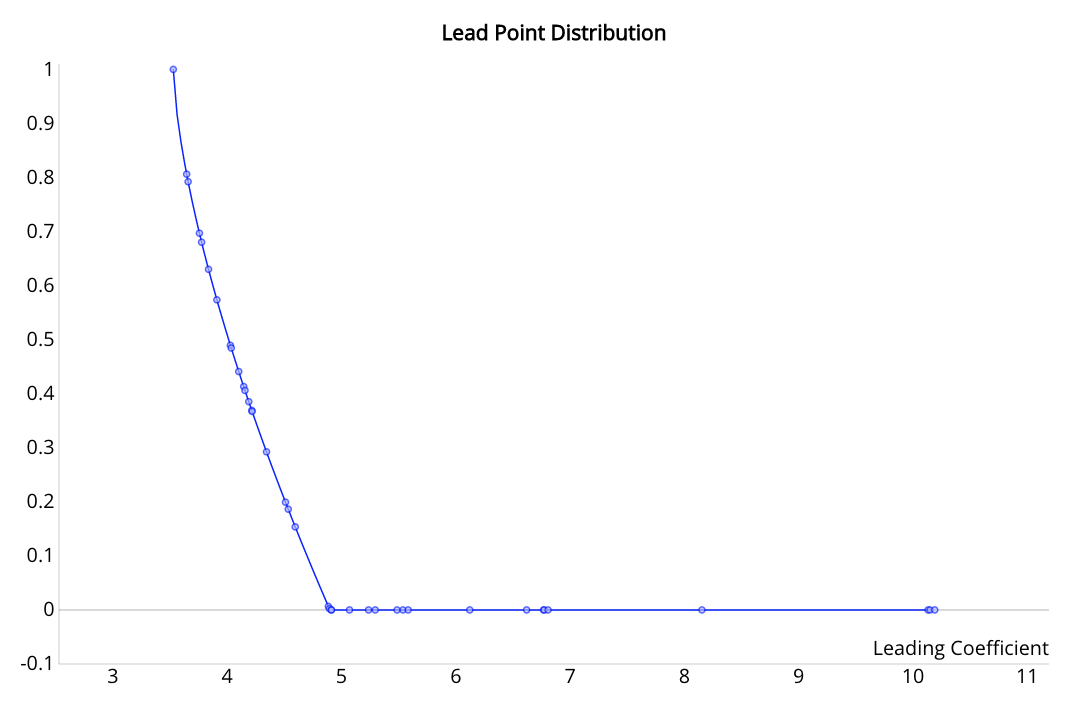Point Fractions Fitting the Curve

March 1, 2019

# Equations for Points

For each aspect of a task flight score there is an equation for points. The independent variable might be something as simple as distance, time, or arrival order or it might be a calculated variable such as leading coefficient or relative difficulty. In all these equations the dependent variable is the fraction of points to be allocated.

Graphing these equations as lines and then overlaying a scatter plot of the fraction of points awarded to each pilot is a good visual check that the scoring was done correctly.

Doing this helped me find bugs I had made not transcribing equations correctly or not bring in all needed inputs.

# Reach a line

Reach is the furthest distance flown irrespective of where the pilot lands or how difficult the course was. The equation for allocating reach points is a simple ratio of distances.

When not including the pilots making it to the end of the speed section, bringing only the distances of pilots that had landed out as inputs to the equation, the scatter plot of awarded fractions for reach points were not lying along the expected line.

BrokenFixed# Time points

I’d made an error in transcribing the above equation for allocating the fraction of time points to each pilot.

BrokenFixed